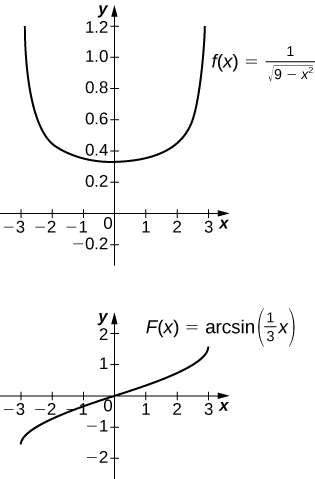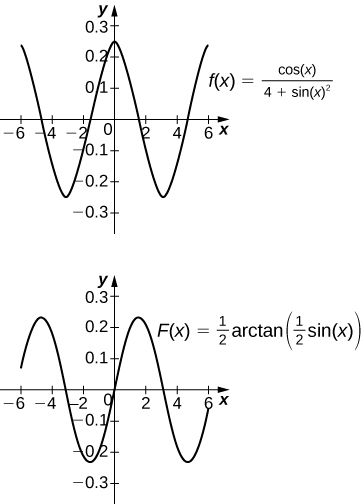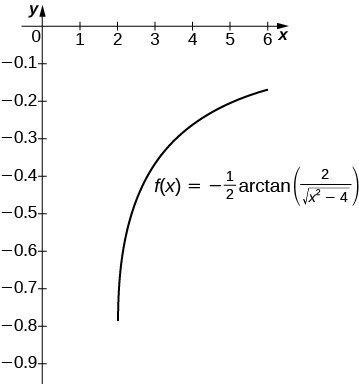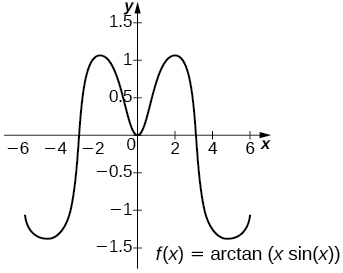# 5.7 Integrals resulting in inverse trigonometric functions  (Page 2/3)

 Page 2 / 3

Use substitution to find the antiderivative of $\int \frac{dx}{25+4{x}^{2}}.$

$\frac{1}{10}\phantom{\rule{0.05em}{0ex}}{\text{tan}}^{-1}\left(\frac{2x}{5}\right)+C$

## Applying the integration formulas

Find the antiderivative of $\int \frac{1}{9+{x}^{2}}dx.$

Apply the formula with $a=3.$ Then,

$\int \frac{dx}{9+{x}^{2}}=\frac{1}{3}\phantom{\rule{0.05em}{0ex}}{\text{tan}}^{-1}\left(\frac{x}{3}\right)+C.$

Find the antiderivative of $\int \frac{dx}{16+{x}^{2}}.$

$\frac{1}{4}\phantom{\rule{0.05em}{0ex}}{\text{tan}}^{-1}\left(\frac{x}{4}\right)+C$

## Evaluating a definite integral

Evaluate the definite integral ${\int }_{\sqrt{3}\text{/}3}^{\sqrt{3}}\frac{dx}{1+{x}^{2}}.$

Use the formula for the inverse tangent. We have

$\begin{array}{}\\ \\ {\int }_{\sqrt{3}\text{/}3}^{\sqrt{3}}\frac{dx}{1+{x}^{2}}\hfill & ={\text{tan}}^{-1}x{|}_{\sqrt{3}\text{/}3}^{\sqrt{3}}\hfill \\ & =\left[{\text{tan}}^{-1}\left(\sqrt{3}\right)\right]-\left[{\text{tan}}^{-1}\left(\frac{\sqrt{3}}{3}\right)\right]\hfill \\ & =\frac{\pi }{6}.\hfill \end{array}$

Evaluate the definite integral ${\int }_{0}^{2}\frac{dx}{4+{x}^{2}}.$

$\frac{\pi }{8}$

## Key concepts

• Formulas for derivatives of inverse trigonometric functions developed in Derivatives of Exponential and Logarithmic Functions lead directly to integration formulas involving inverse trigonometric functions.
• Use the formulas listed in the rule on integration formulas resulting in inverse trigonometric functions to match up the correct format and make alterations as necessary to solve the problem.
• Substitution is often required to put the integrand in the correct form.

## Key equations

• Integrals That Produce Inverse Trigonometric Functions
$\int \frac{du}{\sqrt{{a}^{2}-{u}^{2}}}={\text{sin}}^{-1}\left(\frac{u}{a}\right)+C$
$\int \frac{du}{{a}^{2}+{u}^{2}}=\frac{1}{a}\phantom{\rule{0.05em}{0ex}}{\text{tan}}^{-1}\left(\frac{u}{a}\right)+C$
$\int \frac{du}{u\sqrt{{u}^{2}-{a}^{2}}}=\frac{1}{a}\phantom{\rule{0.05em}{0ex}}{\text{sec}}^{-1}\left(\frac{u}{a}\right)+C$

In the following exercises, evaluate each integral in terms of an inverse trigonometric function.

${\int }_{0}^{\sqrt{3}\text{/}2}\frac{dx}{\sqrt{1-{x}^{2}}}$

${\text{sin}}^{-1}x{|}_{0}^{\sqrt{3}\text{/}2}=\frac{\pi }{3}$

${\int }_{-1\text{/}2}^{1\text{/}2}\frac{dx}{\sqrt{1-{x}^{2}}}$

${\int }_{\sqrt{3}}^{1}\frac{dx}{\sqrt{1+{x}^{2}}}$

${\text{tan}}^{-1}x{|}_{\sqrt{3}}^{1}=-\frac{\pi }{12}$

${\int }_{1\text{/}\sqrt{3}}^{\sqrt{3}}\frac{dx}{1+{x}^{2}}$

${\int }_{1}^{\sqrt{2}}\frac{dx}{|x|\sqrt{{x}^{2}-1}}$

${\text{sec}}^{-1}x{|}_{1}^{\sqrt{2}}=\frac{\pi }{4}$

${\int }_{1}^{2\text{/}\sqrt{3}}\frac{dx}{|x|\sqrt{{x}^{2}-1}}$

In the following exercises, find each indefinite integral, using appropriate substitutions.

$\int \frac{dx}{\sqrt{9-{x}^{2}}}$

${\text{sin}}^{-1}\left(\frac{x}{3}\right)+C$

$\int \frac{dx}{\sqrt{1-16{x}^{2}}}$

$\int \frac{dx}{9+{x}^{2}}$

$\frac{1}{3}\phantom{\rule{0.05em}{0ex}}{\text{tan}}^{-1}\left(\frac{x}{3}\right)+C$

$\int \frac{dx}{25+16{x}^{2}}$

$\int \frac{dx}{|x|\sqrt{{x}^{2}-9}}$

$\frac{1}{3}\phantom{\rule{0.05em}{0ex}}{\text{sec}}^{-1}\left(\frac{x}{3}\right)+C$

$\int \frac{dx}{|x|\sqrt{4{x}^{2}-16}}$

Explain the relationship $\text{−}{\text{cos}}^{-1}t+C=\int \frac{dt}{\sqrt{1-{t}^{2}}}={\text{sin}}^{-1}t+C.$ Is it true, in general, that ${\text{cos}}^{-1}t=\text{−}{\text{sin}}^{-1}t?$

$\text{cos}\left(\frac{\pi }{2}-\theta \right)=\text{sin}\phantom{\rule{0.1em}{0ex}}\theta .$ So, ${\text{sin}}^{-1}t=\frac{\pi }{2}-{\text{cos}}^{-1}t.$ They differ by a constant.

Explain the relationship ${\text{sec}}^{-1}t+C=\int \frac{dt}{|t|\sqrt{{t}^{2}-1}}=\text{−}{\text{csc}}^{-1}t+C.$ Is it true, in general, that ${\text{sec}}^{-1}t=\text{−}{\text{csc}}^{-1}t?$

Explain what is wrong with the following integral: ${\int }_{1}^{2}\frac{dt}{\sqrt{1-{t}^{2}}}.$

$\sqrt{1-{t}^{2}}$ is not defined as a real number when $t>1.$

Explain what is wrong with the following integral: ${\int }_{-1}^{1}\frac{dt}{|t|\sqrt{{t}^{2}-1}}.$

In the following exercises, solve for the antiderivative $\int f$ of f with $C=0,$ then use a calculator to graph f and the antiderivative over the given interval $\left[a,b\right].$ Identify a value of C such that adding C to the antiderivative recovers the definite integral $F\left(x\right)={\int }_{a}^{x}f\left(t\right)dt.$

[T] $\int \frac{1}{\sqrt{9-{x}^{2}}}dx$ over $\left[-3,3\right]$The antiderivative is ${\text{sin}}^{-1}\left(\frac{x}{3}\right)+C.$ Taking $C=\frac{\pi }{2}$ recovers the definite integral.

[T] $\int \frac{9}{9+{x}^{2}}dx$ over $\left[-6,6\right]$

[T] $\int \frac{\text{cos}\phantom{\rule{0.1em}{0ex}}x}{4+{\text{sin}}^{2}x}dx$ over $\left[-6,6\right]$The antiderivative is $\frac{1}{2}\phantom{\rule{0.05em}{0ex}}{\text{tan}}^{-1}\left(\frac{\text{sin}\phantom{\rule{0.1em}{0ex}}x}{2}\right)+C.$ Taking $C=\frac{1}{2}\phantom{\rule{0.05em}{0ex}}{\text{tan}}^{-1}\left(\frac{\text{sin}\left(6\right)}{2}\right)$ recovers the definite integral.

[T] $\int \frac{{e}^{x}}{1+{e}^{2x}}dx$ over $\left[-6,6\right]$

In the following exercises, compute the antiderivative using appropriate substitutions.

$\int \frac{{\text{sin}}^{-1}tdt}{\sqrt{1-{t}^{2}}}$

$\frac{1}{2}{\left({\text{sin}}^{-1}t\right)}^{2}+C$

$\int \frac{dt}{{\text{sin}}^{-1}t\sqrt{1-{t}^{2}}}$

$\int \frac{{\text{tan}}^{-1}\left(2t\right)}{1+4{t}^{2}}dt$

$\frac{1}{4}{\left({\text{tan}}^{-1}\left(2t\right)\right)}^{2}$

$\int \frac{t{\text{tan}}^{-1}\left({t}^{2}\right)}{1+{t}^{4}}dt$

$\int \frac{{\text{sec}}^{-1}\left(\frac{t}{2}\right)}{|t|\sqrt{{t}^{2}-4}}dt$

$\frac{1}{4}\left({\text{sec}}^{-1}{\left(\frac{t}{2}\right)}^{2}\right)+C$

$\int \frac{t{\text{sec}}^{-1}\left({t}^{2}\right)}{{t}^{2}\sqrt{{t}^{4}-1}}dt$

In the following exercises, use a calculator to graph the antiderivative $\int f$ with $C=0$ over the given interval $\left[a,b\right].$ Approximate a value of C , if possible, such that adding C to the antiderivative gives the same value as the definite integral $F\left(x\right)={\int }_{a}^{x}f\left(t\right)dt.$

[T] $\int \frac{1}{x\sqrt{{x}^{2}-4}}dx$ over $\left[2,6\right]$The antiderivative is $\frac{1}{2}\phantom{\rule{0.05em}{0ex}}{\text{sec}}^{-1}\left(\frac{x}{2}\right)+C.$ Taking $C=0$ recovers the definite integral over $\left[2,6\right].$

[T] $\int \frac{1}{\left(2x+2\right)\sqrt{x}}dx$ over $\left[0,6\right]$

[T] $\int \frac{\left(\text{sin}\phantom{\rule{0.1em}{0ex}}x+x\phantom{\rule{0.1em}{0ex}}\text{cos}\phantom{\rule{0.1em}{0ex}}x\right)}{1+{x}^{2}{\text{sin}}^{2}x}dx$ over $\left[-6,6\right]$The general antiderivative is ${\text{tan}}^{-1}\left(x\phantom{\rule{0.1em}{0ex}}\text{sin}\phantom{\rule{0.1em}{0ex}}x\right)+C.$ Taking $C=\text{−}{\text{tan}}^{-1}\left(6\phantom{\rule{0.1em}{0ex}}\text{sin}\left(6\right)\right)$ recovers the definite integral.

find integration of loge x
find the volume of a solid about the y-axis, x=0, x=1, y=0, y=7+x^3
how does this work
Can calculus give the answers as same as other methods give in basic classes while solving the numericals?
log tan (x/4+x/2)
Rohan
Rohan
y=(x^2 + 3x).(eipix)
Claudia
Ismael
A Function F(X)=Sinx+cosx is odd or even?
neither
David
Neither
Lovuyiso
f(x)=1/1+x^2 |=[-3,1]
apa itu?
fauzi
determine the area of the region enclosed by x²+y=1,2x-y+4=0
Hi
MP
Hi too
Vic
hello please anyone with calculus PDF should share
Which kind of pdf do you want bro?
Aftab
hi
Abdul
can I get calculus in pdf
Abdul
How to use it to slove fraction
Hello please can someone tell me the meaning of this group all about, yes I know is calculus group but yet nothing is showing up
Shodipo
You have downloaded the aplication Calculus Volume 1, tackling about lessons for (mostly) college freshmen, Calculus 1: Differential, and this group I think aims to let concerns and questions from students who want to clarify something about the subject. Well, this is what I guess so.
Jean
Im not in college but this will still help
nothing
how can we scatch a parabola graph
Ok
Endalkachew
how can I solve differentiation?
with the help of different formulas and Rules. we use formulas according to given condition or according to questions
CALCULUS
For example any questions...
CALCULUS
v=(x,y) وu=(x,y ) ∂u/∂x* ∂x/∂u +∂v/∂x*∂x/∂v=1
log tan (x/4+x/2)
Rohan
what is the procedures in solving number 1?
review of funtion role?
for the function f(x)={x^2-7x+104 x<=7 7x+55 x>7' does limx7 f(x) exist?
no
Haliru
is derivatives of any constant no is zero
Haliru
find dy÷dx (y^2+2 sec)^2=4(x+1)^2
Integral of e^x/(1+e^2x)tan^-1 (e^x)By Miranda ReisingBy Cameron CaseyBy OpenStaxBy Miranda ReisingBy David GeltnerByBy Tess ArmstrongBy OpenStaxBy Rachel WoolardBy Stephen Voron